MORE IN Network Analysis
Total marks: --
Total time: --
INSTRUCTIONS
(1) Assume appropriate data and state your reasons
(2) Marks are given to the right of every question
(3) Draw neat diagrams wherever necessary

1 (a) The node equations of a network are $\left [ \dfrac {1}{5}+ \dfrac {1}{2}j + \dfrac {1}{4} \right ]V_1 - \dfrac {1}{4}V_2 = \dfrac {50 \angle 06^\circ}{5} \ and \ -\dfrac {1}{4} V_1 + \left [ \dfrac {1}{4}- \dfrac {1}{2j}+ \dfrac {1}{2} \right ]v_2 = \dfrac {50 \angle 906^\circ}{2}$
10 M
1 (b) Find the current 1 in 28Ω resistor by mesh analysis in Fig. Q1 (b)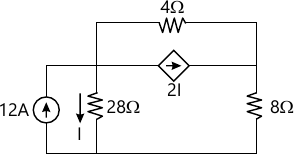5 M
1 (c) Using source transformation find power delivered by 50 V source in given network of Fig Q1 (c).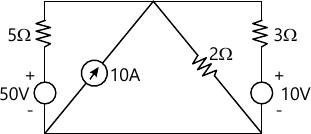5 M

2 (a) Define the following terms with respect to network topology and give example:
i) Oriented and unoriented graphs.
ii) Isomorphic graphs
iii) Fundamental cut set.
6 M
2 (b) For the network shown in Fig Q2(b), write the tie set schedule selecting centre star as tree and find all the branch currents by solving equilibrium equations.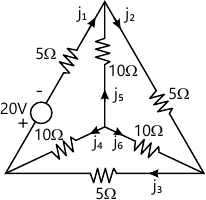10 M
2 (c) For the network shown in Fig Q2(c) draw the dual network.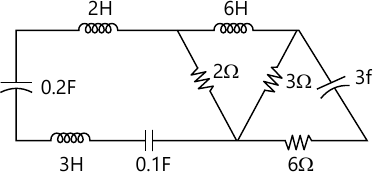4 M

3 (a) State and prove superposition theorem.
6 M
3 (b) Find i8 and hence verify reciprocity theorem for the network in Fig Q3 (b).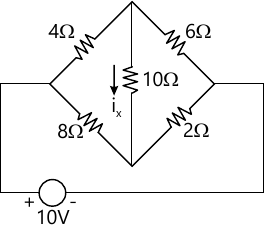8 M
3 (c) Using Millman's theorem find IL through RL for the network of Fig. Q3 (c).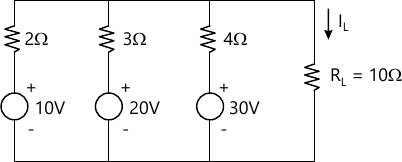6 M

4 (a) State and prove Thevenin's theorem.
6 M
4 (b) Find the value of load resistance when maximum power is transfered across it and also find the value of maximum power transferred for the network of Fig. Q4(b).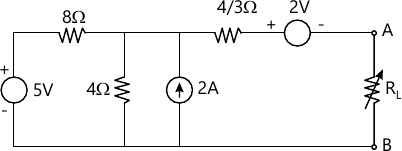8 M
4 (c) Find the current through 16Ω resistor using Norton's theorem in Fig. Q4(c).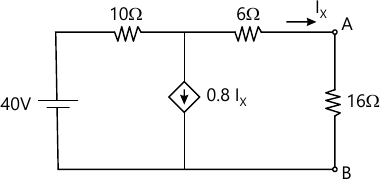6 M

5 (a) Define the following terms i) Resonance
ii) Q-Factor
iii) Selectivity of series RIC circuit
iv) Bandwidth
4 M
5 (b) Prove that $f_0 = \sqrt{f_1, f_2}$ where f1 and f2 are the two half power frequencies of a resonant circuits.
8 M
5 (c) A series RLC circuit has R=4Ω, L=1mH and C=10 μF. Calculate Q factor, band width resonant frequency and the half power frequencies f1 and f2.
8 M

6 (a) For the circuit shown in Fig Q6 (a), determine complete solution for current when switch K is closed at t=0. Applied voltage is y(t) which is given as $100\cos \left (10^3 t + \dfrac {\pi}{2} \right )$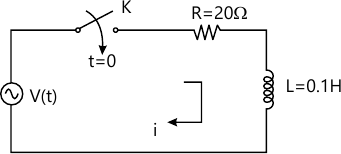10 M
6 (b) For the given circuit of Fig. Q 6(b) switch K is changed from position 1 to position 2 at t=0, the steady having been reached before switching. Find the value of$i, \dfrac {di}{dt} \ and \ \dfrac {d^2i}{dt^2}$ at t=0^- \]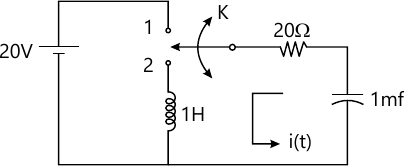10 M

7 (a) State and prove initial value and final value theorem.
8 M
7 (b) Obtain the Laplace transform of the saw tooth waveform shown in Fig. Q7(b).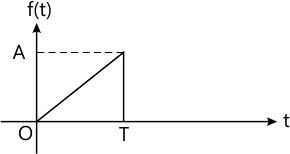8 M
7 (c) Find the Laplace transform of (i) t (ii) Δ (t)
4 M

8 (a) Obtain the relationship between h and y parameters of a two port network.
8 M
8 (b) Determine the transmission parameters for the network shown in fig Q8 (b).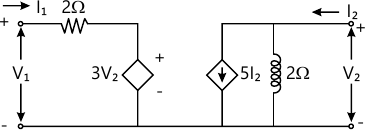8 M
8 (c) Define z parameter and draw the equivalent network in terms of z parameters.
4 M

More question papers from Network Analysis